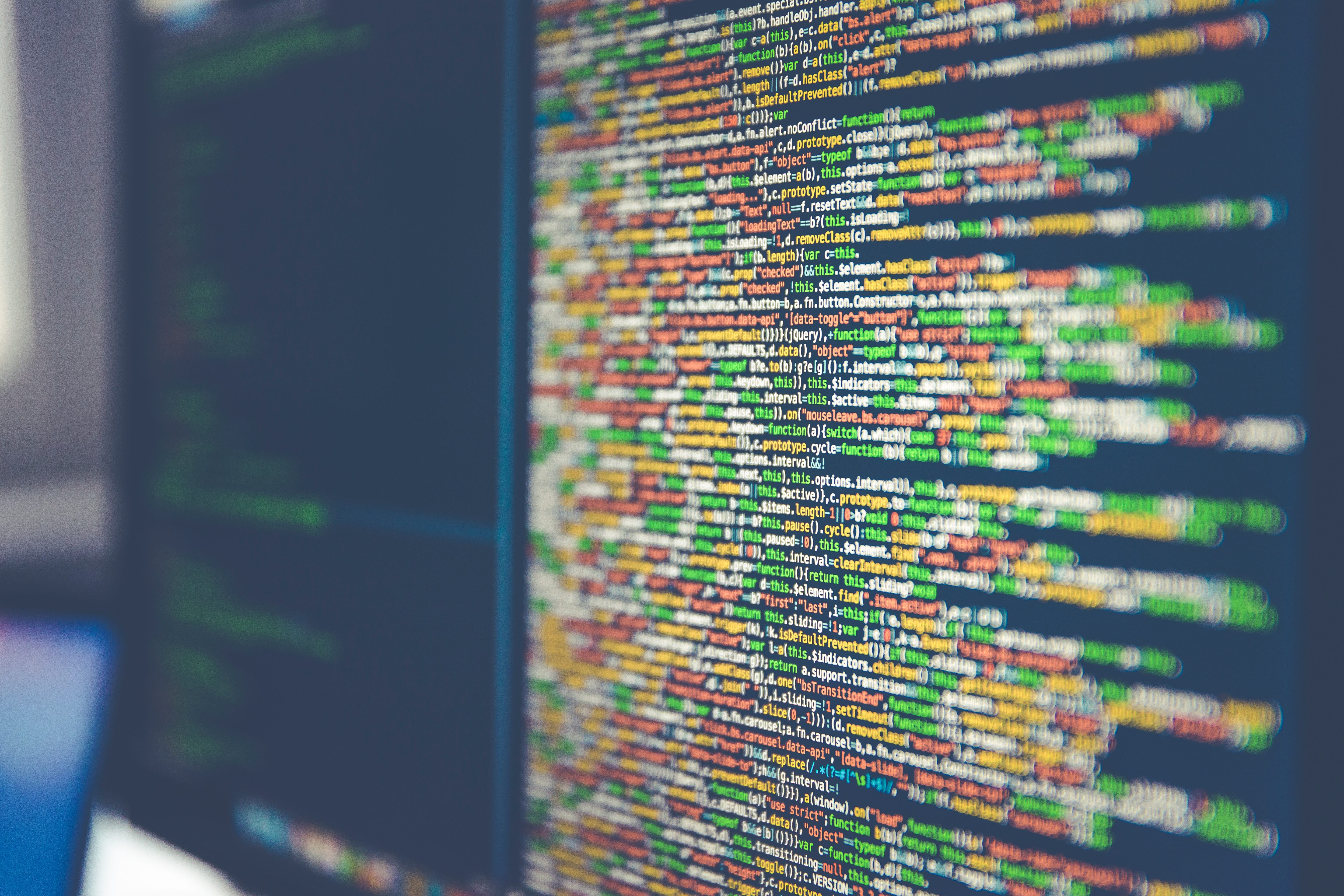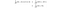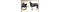Photo by Markus Spiske

# QC — What are Qubits in Quantum computing?

Qubits are the core component in quantum computing. With superposition, we can encode an exponential amount of information that can scale a solution better than classical computing. In Part 1, we take a look at the motivation for quantum computing and study some quantum mechanics principles. In part 2 here, we go into the core of quantum computing and demystify the concept of qubits.

# Superposition

The concept of superposition is important because this is what qubits (quantum bits) about! So let’s start developing a mathematical model for the spin. Don’t get scared, it is pretty simple. The pace will be slower in this section so you can take time to adjust to the notation in quantum mechanics. It builds the foundation of something pretty fun. Also, the math is far more simple than reconciling the theory with intuition.SourceSource

# Next

Now, we understand the Qubits, the equivalent of bits in the classical computer but far more powerful. In a classical computer, we have +, -, ×, ÷ operators to manipulate bits. Quantum computers have none of them. So how do we manipulate qubits? This will be answered in the following article.